# Measuring Line Parameters with an LETG/ACIS-S Spectrum## Overview

#### Synopsis:

After having created or downloaded a set of PHA2 and response files (RMFs and ARFs) for a LETG/ACIS-S observation, perform a simple fit to several of the line features present in the spectra. This thread takes the user through a calculation of the error bars on the line normalizations, positions, and widths, and shows how to calculate the line equivalent widths.

For those wishing to perform their analysis with XSPEC, the PHA2 file must first be split into individual type 1 PHA files, and then grouped to increase the signal-to-noise in each spectral bin. The Grouping a Grating Spectrum CIAO thread shows how to do this, and the procedure is also included within this thread.

You are working with a LETG/ACIS-S data set, and want to begin a simple analysis of the spectrum.

Last Update: 8 Dec 2022 - updated for CIAO 4.15, typos corrected.

## Data Preparation

This thread makes use of archival data products for the star σ Geminorum (σ Gem for short), downloaded from TGcat. They were obtained by performing a "Search by ObsID" for ObsID 613, then choosing "viewfile table" for the target, and clicking the download arrow next to each filename. These files were unzipped and placed in the directory in which the data analysis is to be performed.

Note that we are not considering any background files. For LETG/ACIS-S observations the background is suppressed by the process of order sorting. That is, an event at a given location along the grating arms must fall into a narrow range of energies (as determined by the CCD) in order for it to be considered a valid dispersed photon. Most background events do not meet these "order sorting" criteria. For this and many other LETG/ACIS-S observations, the first simple analysis can be performed without reference to the background. (Detailed analysis of low signal-to-noise spectra likely would require proper consideration of the background. See the Fitting Grating Data thread for an example of analysis including background data.)

Those using either Sherpa or ISIS to perform their analysis can use the data files directly in the analysis; skip to the "Load the spectrum and responses" section.

XSPEC users, however, must split and group the spectra before the analysis as described here.

## Load the spectrum and responses

Start a Sherpa session:

```unix% sherpa
-----------------------------------------------------
Welcome to Sherpa: CXC's Modeling and Fitting Package
-----------------------------------------------------
CIAO 4.15 Sherpa version 1 Thursday, October 13, 2022

Python 3.9.9 (main, Mar 24 2022, 22:02:45)
IPython 8.0.0 -- An enhanced Interactive Python. Type '?' for help.

IPython profile: sherpa
Using matplotlib backend: TkAgg

sherpa>
```

We begin by reading the PHA2 file that contains the grating spectra. This file contains 6 spectra, 3 spectral orders each for both positive and negative order LETG spectra. They are stored in the order: -3/-2/-1/1/2/3. If no filter is used when loading the data, then Sherpa will read in all 6 rows in order, assigning them to datasets 1-6.

We are only interested, however, in the first-order spectra (positive and negative); therefore, we use a Data Model-type filter to select the third and fourth rows of the PHA2 file (LETG -1 and LETG +1, respectively).

```sherpa> load_pha("pha2[#row=3,4]")
statistical errors were found in file 'pha2[#row=3,4]'
but not used; to use them, re-read with use_errors=True
read background_up into a dataset from file pha2[#row=3,4]
read background_down into a dataset from file pha2[#row=3,4]
Multiple data sets have been input: 1-2
```Note

Alternatively, one could use a filter to select all negative and postitive first-order spectra via the TG_M keyword:

```sherpa> load_pha("pha2[TG_M=-1,1]")
```

Note that associated background spectra (two each for each spectrum, one from either side of the gratings arm) will be read in with the source spectra. However, unless the subtract command is called, or unless one creates a background model, they will not be part of the fitting process. For the remainder of this thread, we ignore these background spectra.

We now read in the associated ARF files and assign them to their corresponding datasets, then do the same for the RMF files.

```sherpa> load_arf(1, "leg_-1.arf")

```

## Group and filter the data

Sherpa allows one to bin the spectra during the analysis session; see the Sherpa thread Changing the grouping scheme of a data set within Sherpa for details. We bin each of the spectra to have a minimum of 20 counts per energy channel.

```sherpa> group_counts(1, 20)
sherpa> group_counts(2, 20)
```

For the purposes of this analysis, we will restrict ourselves to only fitting the lines in the 1.4-2.2 keV region. (As we shall see below, this region alone shows multiple lines.) We restrict the energy range to 1.4-2.2 keV keV for both spectra using the notice command.

```sherpa> print(get_analysis(1))
energy

sherpa> notice(1.4, 2.2)
dataset 1: 0.119907:12.3984 -> 1.39898:2.20416 Energy (keV)
dataset 2: 0.119907:12.3984 -> 1.39898:2.20416 Energy (keV)
```

The spectra can all be plotted in the same figure using the plot command (Figure 1):

```sherpa> plot("data", 1, "data", 2)
sherpa> plt.savefig('all.png')
```

### Figure 1: Plot of the +1 and -1 LETG spectra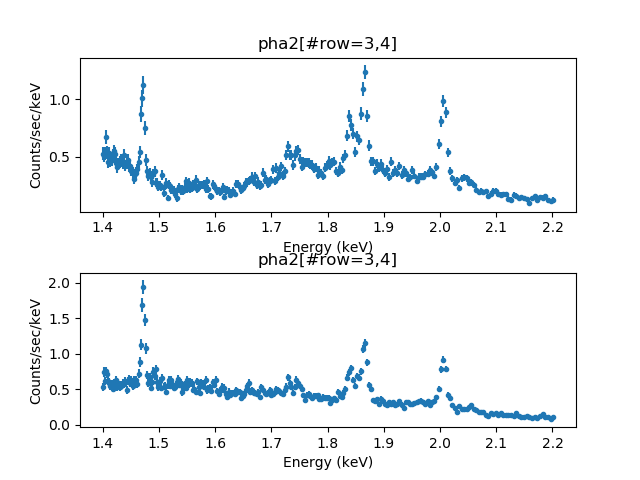### Figure 1: Plot of the +1 and -1 LETG spectra

The -1 LETG order is in the upper plot and the +1 LETG order is in the lower plot. The plots have been filtered to the range 1.4-2.2 keV.

## Defining the Source Models

We will first fit a broad continuum to the spectra, without any lines. The XSPEC power-law model (xspowerlaw) is chosen for the continuum. It is assigned as the source for data set 1 and given the model name "powr"; the model name is then used to assign the same source model to data set 2.

```sherpa> set_source(1, xspowerlaw.powr)
sherpa> set_source(2, powr)

sherpa> show_source()
Model: 1
xspowerlaw.powr
Param        Type          Value          Min          Max      Units
-----        ----          -----          ---          ---      -----
powr.PhoIndex thawed            1           -3           10
powr.norm    thawed            1            0        1e+24

Model: 2
xspowerlaw.powr
Param        Type          Value          Min          Max      Units
-----        ----          -----          ---          ---      -----
powr.PhoIndex thawed            1           -3           10
powr.norm    thawed            1            0        1e+24
```

We can see that the starting point of the fit is not ideal, so we manually adjust the power-law normalization by eye (estimating a factor of 100 difference from the plot_fit result), and overplot the new starting plot with plot_model (resulting in Figure 2):

```sherpa> plot_fit(1, ylog=True)
sherpa> powr.norm = 0.01
sherpa> plot_model(1, overplot=True)
```

### Figure 2: Before the fit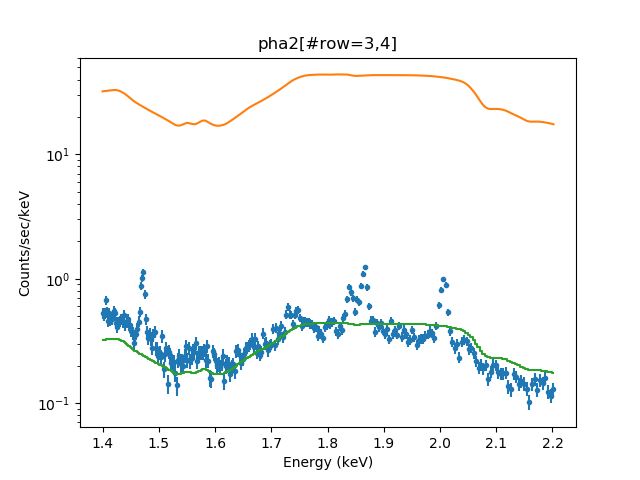### Figure 2: Before the fit

The orange line shows the original model—when the norm was set to 1—while the green line shows the model after reducing the normlization by a factor of 100.

## Fitting

The fit statistic is set to be χ2 with the data counts acting as the variance (i.e., a Gaussian approximation to simple Poisson statistics). Both data sets are fit simultaneously.

```sherpa> set_stat("chi2datavar")
sherpa> fit()
WARNING: data set 1 has associated backgrounds, but they have not been subtracted, nor have background models been set
WARNING: data set 2 has associated backgrounds, but they have not been subtracted, nor have background models been set
Datasets              = 1, 2
Method                = levmar
Statistic             = chi2datavar
Initial fit statistic = 6941.83
Final fit statistic   = 4371.46 at function evaluation 19
Data points           = 518
Degrees of freedom    = 516
Probability [Q-value] = 0
Reduced statistic     = 8.47182
Change in statistic   = 2570.37
powr.PhoIndex   2.73878      +/- 0.0338374
powr.norm      0.028444     +/- 0.000538873
```Note

In this particular case, changing the normalization before the fit doen't change the final fit results. It can be useful in more complicated cases (although care should be taken to ensure that the fit has not found a local, rather than global, minimum).

At any time, the results of the last fit can be displayed using the show_fit command. The fit yields the following results:

```sherpa> show_fit()
Optimization Method: LevMar
name     = levmar
ftol     = 1.1920928955078125e-07
xtol     = 1.1920928955078125e-07
gtol     = 1.1920928955078125e-07
maxfev   = None
epsfcn   = 1.1920928955078125e-07
factor   = 100.0
numcores = 1
verbose  = 0

Statistic: Chi2DataVar
Chi Squared with data variance.

The variance in each bin is estimated from the data value in that
bin.

If the number of counts in each bin is large, then the shape of
the Poisson distribution from which the counts are sampled tends
asymptotically towards that of a Gaussian distribution, with
variance

sigma(i)^2 = N(i,S) + [A(S)/A(B)]^2 N(i,B)

where N is the number of on-source (and off-source) bins included
in the fit. The background term appears only if an estimate of the
background has been subtracted from the data.

A(B) is the off-source "area", which could be
the size of the region from which the background is extracted, or
the length of a background time segment, or a product of the two,
etc.; and A(S) is the on-source "area". These terms may be defined
for a particular type of data: for example, PHA data sets A(B) to
`BACKSCAL * EXPOSURE` from the background data set and A(S) to
`BACKSCAL * EXPOSURE` from the source data set.

--------
Chi2Gehrels, Chi2ModVar, Chi2XspecVar

Fit:Datasets              = 1, 2
Method                = levmar
Statistic             = chi2datavar
Initial fit statistic = 6941.83
Final fit statistic   = 4371.46 at function evaluation 19
Data points           = 518
Degrees of freedom    = 516
Probability [Q-value] = 0
Reduced statistic     = 8.47182
Change in statistic   = 2570.37
powr.PhoIndex   2.73878      +/- 0.0338374
powr.norm      0.028444     +/- 0.000538873
```

The results of these fits is shown in Figure 3:

```sherpa> plot("fit", 1, "fit", 2)
```

### Figure 3: Continuum fit to the data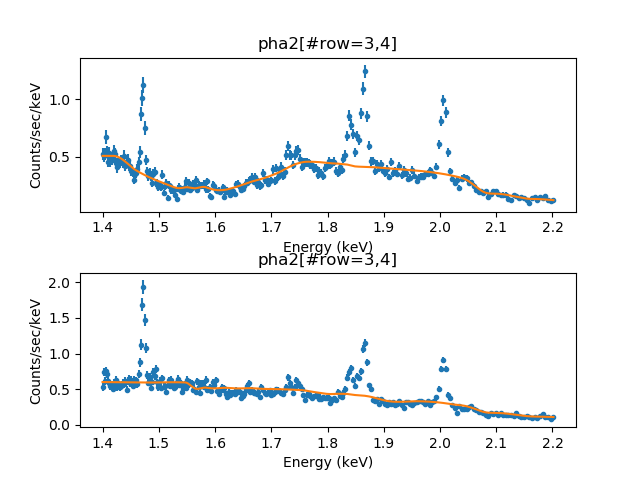### Figure 3: Continuum fit to the data

The -1 LETG order is in the upper plot and the +1 LETG order is in the lower plot.

## Adding lines to the model and fitting again

We start by creating a Gaussian model component for each of these features, and assign them to names indicative of their general energy location. We set each of their initial line widths to be narrow (i.e., 10-3), their line normalizations to be small (10-4), and their line energies to be close to the observed features. Additionally, to prevent the lines from broadening during the initial fits, we initially freeze the line widths.

The create_model_component command is used to establish each model component before setting the complex source expressions.

```sherpa> create_model_component("xsgaussian", "g15")
<XSgaussian model instance 'xsgaussian.g15'>
sherpa> g15.norm = 1.e-4
sherpa> g15.sigma = 1.e-3
sherpa> g15.linee = 1.47

sherpa> create_model_component("xsgaussian", "g18a")
<XSgaussian model instance 'xsgaussian.g18a'>
sherpa> g18a.norm = 1.e-4
sherpa> g18a.sigma = 1.e-3
sherpa> g18a.linee = 1.84

sherpa> create_model_component("xsgaussian", "g18b")
<XSgaussian model instance 'xsgaussian.g18b'>
sherpa> g18b.norm = 1.e-4
sherpa> g18b.sigma = 1.e-3
sherpa> g18b.linee = 1.87

sherpa> create_model_component("xsgaussian", "g2")
<XSgaussian model instance 'xsgaussian.g2'>
sherpa> g2.norm = 1.e-4
sherpa> g2.sigma = 1.e-3
sherpa> g2.linee = 2
```

The sigma parameter of each model, which is the line width, is frozen before the fit:

```sherpa> freeze(g15.sigma, g18a.sigma, g18b.sigma, g2.sigma)
```

We create a model with a power-law continuum plus these four line components, examine the source definition for one of the data sets (they are identical).

```sherpa> full_model = powr + g15 + g18a + g18b + g2
sherpa> set_source(1, full_model)
sherpa> set_source(2, full_model)

sherpa> show_source(1)
Model: 1
((((xspowerlaw.powr + xsgaussian.g15) + xsgaussian.g18a) + xsgaussian.g18b) + xsgaussian.g2)
Param        Type          Value          Min          Max      Units
-----        ----          -----          ---          ---      -----
powr.PhoIndex thawed      2.73878           -3           10
powr.norm    thawed     0.028444            0        1e+24
g15.LineE    thawed         1.47            0        1e+06        keV
g15.Sigma    frozen        0.001            0           20        keV
g15.norm     thawed       0.0001            0        1e+24
g18a.LineE   thawed         1.84            0        1e+06        keV
g18a.Sigma   frozen        0.001            0           20        keV
g18a.norm    thawed       0.0001            0        1e+24
g18b.LineE   thawed         1.87            0        1e+06        keV
g18b.Sigma   frozen        0.001            0           20        keV
g18b.norm    thawed       0.0001            0        1e+24
g2.LineE     thawed            2            0        1e+06        keV
g2.Sigma     frozen        0.001            0           20        keV
g2.norm      thawed       0.0001            0        1e+24
```

The starting point for the fit (shown just for the LEG -1 order) is shown in Figure 4:

```sherpa> plot_fit_delchi(1)
```

### Figure 4: Starting point for the line fit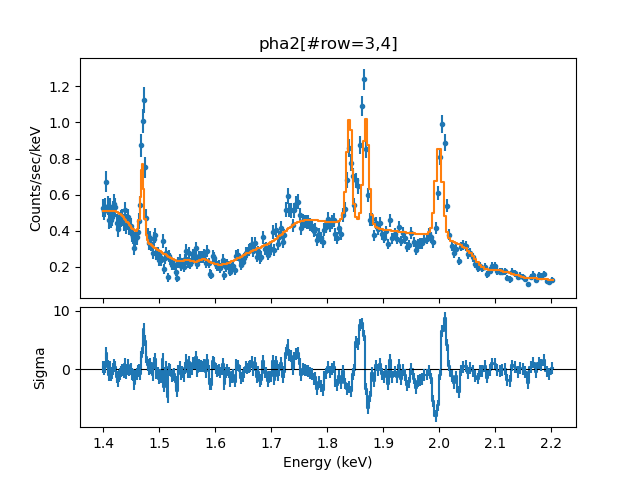### Figure 4: Starting point for the line fit

Let's see how the fit does to reduce the structure in the residuals!

It is time to fit the data (at least, the line centroids and amplitudes):

```sherpa> fit()
WARNING: data set 1 has associated backgrounds, but they have not been subtracted, nor have background models been set
WARNING: data set 2 has associated backgrounds, but they have not been subtracted, nor have background models been set
Datasets              = 1, 2
Method                = levmar
Statistic             = chi2datavar
Initial fit statistic = 2423.51
Final fit statistic   = 871.452 at function evaluation 93
Data points           = 518
Degrees of freedom    = 508
Probability [Q-value] = 1.40606e-21
Reduced statistic     = 1.71546
Change in statistic   = 1552.06
powr.PhoIndex   2.92076      +/- 0.0371845
powr.norm      0.0294724    +/- 0.000604278
g15.LineE      1.47194      +/- 0.000130228
g15.norm       0.000185117  +/- 5.97299e-06
g18a.LineE     1.84039      +/- 0.000251236
g18a.norm      7.23642e-05  +/- 3.4542e-06
g18b.LineE     1.86453      +/- 0.000145066
g18b.norm      0.000138762  +/- 4.0664e-06
g2.LineE       2.00539      +/- 0.000550119
g2.norm        0.000125724  +/- 3.95501e-06
```

Let's look at how the residuals look (Figure 5):

```sherpa> plot_delchi(1)
sherpa> plot_delchi(2, overplot=True)
sherpa> for mdl in [g15, g18a, g18b, g2]:
...:    plt.axvline(mdl.lineE.val, color='black', linewidth=2)
...:
```

### Figure 5: Residuals from the line fit (with fixed widths)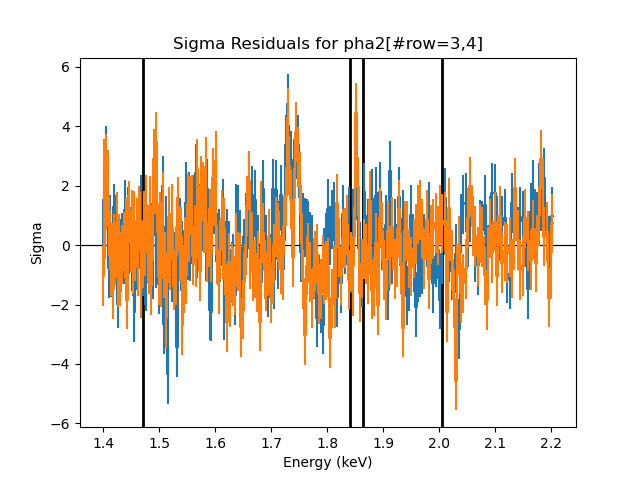### Figure 5: Residuals from the line fit (with fixed widths)

The blue points are from dataset 1 (-1 order) and the orange points from dataset 2 (+1 order). Four black vertical lines mark the line positions. Note that when looping over each line, the line position was accessed as 'mdl.lineE.val' in order to use the numeric value of the parameter.

Now that most of our parameters are close to their final values and we have a good description of the lines, we thaw the line widths, and then refit the data.

```sherpa> thaw(g15.sigma, g18a.sigma, g18b.sigma, g2.sigma)
sherpa> fit()
WARNING: data set 1 has associated backgrounds, but they have not been subtracted, nor have background models been set
WARNING: data set 2 has associated backgrounds, but they have not been subtracted, nor have background models been set
Datasets              = 1, 2
Method                = levmar
Statistic             = chi2datavar
Initial fit statistic = 871.452
Final fit statistic   = 856.993 at function evaluation 266
Data points           = 518
Degrees of freedom    = 504
Probability [Q-value] = 9.89181e-21
Reduced statistic     = 1.70038
Change in statistic   = 14.4598
powr.PhoIndex   2.93225      +/- 0.0380361
powr.norm      0.0295877    +/- 0.000617883
g15.LineE      1.47194      +/- 0.000136657
g15.Sigma      0.000996139  +/- 0.000456943
g15.norm       0.000185178  +/- 6.39705e-06
g18a.LineE     1.84084      +/- 0.000404948
g18a.Sigma     0.00366954   +/- 0.000663747
g18a.norm      7.82145e-05  +/- 4.37247e-06
g18b.LineE     1.86456      +/- 0.000219171
g18b.Sigma     0.00256177   +/- 0.000464499
g18b.norm      0.00014157   +/- 4.66663e-06
g2.LineE       2.00486      +/- 0.623155
g2.Sigma       0.000608589  +/- 0.136176
g2.norm        0.000125959  +/- 4.27202e-06
```

The four fitted lines correspond to Mg XII, two lines of Si XIII, and Si XIV. The fitted line energies all lie within 40-130 km/sec of their expected rest energies. (One can check for the expected line energies, for example, using the Interactive Guide for ATOMDB, the atomic line database.) Below, we will return to the question of: to what extent are these small deviations significant?

We can now plot these fitted spectra, and look at the δχ residuals of the fit (Figure 6):

```sherpa> plot("fit", 1, "fit", 2, "delchi", 1, "delchi", 2)
```

### Figure 6: Fit and residuals to the spectral lines: widths free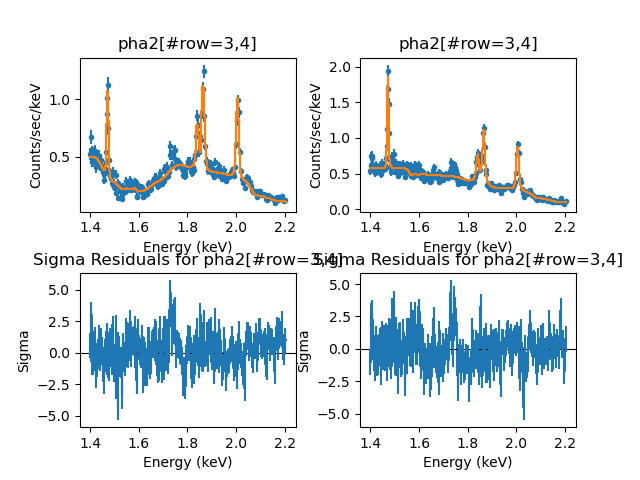### Figure 6: Fit and residuals to the spectral lines: widths free

The -1 LETG order is in the left half of the plot and the +1 LETG order is in the right half of the plot.

## Examine the fit results

In order to explore the errors on the line parameters, we can now use the conf() command to run the Sherpa confidence routines. As is common in X-ray astronomy, we search for the 90% confidence level values for one interesting parameter (i.e., the variation of the parameter of interest that when refitting the remaining unfrozen parameters yields a change in the χ2 value of 2.706). This corresponds to an error bar of 1.64σ (1σ being the 68% confidence interval), and set this as the default error value in the projection routines via the set_conf_opt command. We then run conf for the three parameters of each of the Gaussian model components: line energy, line width, and line normalization.

```sherpa> set_conf_opt("sigma", 1.64)
sherpa> pars = []
sherpa> for mdl in [g15, g18a, g18b, g2]:
...: pars.extend([mdl.linee, mdl.sigma, mdl.norm])
...:
sherpa> conf(*pars)
WARNING: data set 1 has associated backgrounds, but they have not been subtracted, nor have background models been set
WARNING: data set 2 has associated backgrounds, but they have not been subtracted, nor have background models been set
g18b.LineE lower bound:	-0.000392235
g18a.LineE lower bound:	-0.000724582
g15.LineE -: WARNING: The confidence level lies within (1.471676e+00, 1.471809e+00)
g15.LineE lower bound:	-0.000198284
g2.LineE -: WARNING: The confidence level lies within (2.002362e+00, 2.004863e+00)
g2.LineE lower bound:	-0.00125012
g15.LineE upper bound:	0.000216873
g18a.LineE upper bound:	0.000824829
g18b.LineE upper bound:	0.00038687
g18a.Sigma lower bound:	-0.00139014
g18b.Sigma lower bound:	-0.00211267
g15.Sigma -: WARNING: The confidence level lies within (9.331418e-05, 9.485751e-05)
g15.Sigma lower bound:	-0.000902053
g18a.Sigma upper bound:	0.00130641
g15.Sigma upper bound:	0.000598113
g18b.Sigma upper bound:	0.000837398
g18a.norm lower bound:	-7.51752e-06
g15.norm lower bound:	-1.05409e-05
g18b.norm lower bound:	-8.1578e-06
g15.norm upper bound:	1.07281e-05
g18b.norm upper bound:	8.11589e-06
g18a.norm upper bound:	7.95106e-06
g2.LineE +: WARNING: The confidence level lies within (2.007842e+00, 2.007837e+00)
g2.LineE upper bound:	0.00297636
g2.Sigma lower bound:	-----
g2.Sigma upper bound:	0.00104132
g2.norm lower bound:	-6.53212e-06
g2.norm upper bound:	6.53411e-06
Datasets              = 1, 2
Confidence Method     = confidence
Iterative Fit Method  = None
Fitting Method        = levmar
Statistic             = chi2datavar
confidence 1.64-sigma (89.8995%) bounds:
Param            Best-Fit  Lower Bound  Upper Bound
-----            --------  -----------  -----------
g15.LineE         1.47194 -0.000198284  0.000216873
g15.Sigma     0.000996139 -0.000902053  0.000598113
g15.norm      0.000185178 -1.05409e-05  1.07281e-05
g18a.LineE        1.84084 -0.000724582  0.000824829
g18a.Sigma     0.00366954  -0.00139014   0.00130641
g18a.norm     7.82145e-05 -7.51752e-06  7.95106e-06
g18b.LineE        1.86456 -0.000392235   0.00038687
g18b.Sigma     0.00256177  -0.00211267  0.000837398
g18b.norm      0.00014157  -8.1578e-06  8.11589e-06
g2.LineE          2.00486  -0.00125012   0.00297636
g2.Sigma      0.000608589        -----   0.00104132
g2.norm       0.000125959 -6.53212e-06  6.53411e-06
```

Note that confidence failed to find a lower limit for the g2.Sigma parameter; we shall return to the reasons for this.

For those parameters that did return valid parameter bounds, we see that line energies are determined with accuracies ranging from 0.2 eV to 0.8 eV, which translate to equivalent velocities of 40-130 km/sec. In this energy range, the Half Width Half Maximum (HWHM) resolution of the LETG ranges from 700-900 km/sec. That is, due to the extremely good statistics of these very strong lines, the formal error bars are 7-18 times smaller than HWHM. Whereas it is quite possible with sufficiently good statistics to centroid a Gaussian to better than HWHM, such a large factor of improvement cautions against over-interpretation, and implies that systematic concerns may dominate. For example, if one were to fit the line with a Voigt profile instead of a Gaussian, systematically different results may be obtained. However, given the high signal-to-noise, we would expect that any systematic differences obtained from using alternative line profiles likely would be smaller than HWHM.

The expected energies for these lines are 1.4720 keV (12 km/sec offset), 1.8395 keV (220 km/sec offset), 1.8650 keV (70 km/sec offset) and 2.0049 keV (6 km/sec offset). That is, all are detected at their rest energies to well-within what we would consider to be reasonable systematic uncertainties.

Similar statements can be made about their fitted widths. The fitted widths range over equivalent velocities of 90-600 km/sec. The two broadest lines, i.e., at 1.841 keV and 1.865 keV, could also have a third line in between them (the expected intercombination line at 1.854 keV). At this point, it would be reasonable for the user to explore the limits that one could place on the presence of this line, and how it systematically affects the fits. For example, all three lines could be fit, but with their relative energies fixed to theoretical expectations. Likewise, one also could explore fixing their widths to be the same fractional width (under the assumption that they all come from the same velocity regions).

The line at 2.005 keV had a lower limit line width that reached 0 before a Δχ2 of 2.7 was found; therefore, the upper bound (1.04 eV) reasonably could be considered an upper-limit to the line width. This corresponds to a velocity of 155 km/sec. Given that this is a factor of a few below the HWHM of LETG, it would be prudent to explore the systematic dependence of this value upon model assumptions.

The fact that the line energy and width fit uncertainties are well-below the LETG HWHM resolution gives some indication as to why the confidence algorithm failed to find one of the 24 parameter limits. The confidence algorithm tries to determine the bounds by extrapolating the shape of the χ2 surface near the best-fit value of the parameter, under the assumption that it is relatively "well-behaved". Here, due to the very high signal-to-noise and being at the systematic limits of the detector, some of the χ2 contours are "badly behaved". Here we show a statistic vs. parameter curve for the line energy that failed to converge, by using the int_proj (interval projection) function.

For the line near 2 keV, we explore 50 values of the fit statistic in a narrow bound around the best-fit value (Figure 7):

```sherpa> int_proj(g2.linee, min=2.0037, max=2.00785, nloop=50)
```

### Figure 7: Interval-projection of the line near 2 keV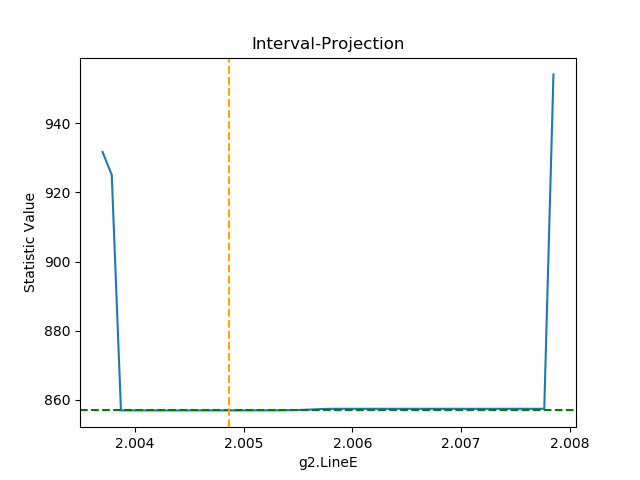### Figure 7: Interval-projection of the line near 2 keV

As can be seen, this statistic curve is very different from quadratic, and has large sudden jumps at boundaries near 2.004 keV and 2.008 keV (i.e., a width with an equivalent velocity of 600 km/sec, comparable to the HWHM of the LETG). Thus, one could trust the results of the confidence method and reasonably use this interval as the bound on the uncertainty of this line energy. Again, one should explore the systematic dependence of this line energy upon model assumptions.

On the other hand, the statistic contours for all the line amplitudes (not shown) are very smooth and nearly quadratic. For these parameters, we expect the results of projection to be a very good estimate of the uncertainties on the line strengths. All the line normalizations are determined to an accuracy of 5-10% at the 90% confidence level for one interesting parameter; however, in terms of systematic effects, one could also add the expected intercombination line at 1.854 keV to see how that line affects the fitted normalizations of the other two (resonance and forbidden) lines near 1.8 keV.

## Calculate the equivalent width of a line

Rather than merely presenting the line normalizations, we can instead calculate the line equivalent width. The line equivalent width is an often used measure for the line strength relative to the underlying continuum. Sherpa has a function for evaluating the equivalent width, eqwidth. The required inputs are the source model absent the line feature of interest and the source model with the line feature of interest.

Optionally, the data set number for which the equivalent width will be calculated can also be given as an input, but here as we apply the same model and parameters to both datasets, this is not necessary. There are also optional lo and hi arguments for restricting the calculation of the equivalent width to a subset of the full energy or wavelength range of a data set.

```sherpa> for mdl in [g15, g18a, g18b, g2]:
...:      ew = 1000 * eqwidth(powr, powr + mdl)
...:      print("Line {:15s}  EW = {:.2f} eV".format(mdl.name, ev))
...:
Line xsgaussian.g15   EW = 19.44 eV
Line xsgaussian.g18a  EW = 15.82 eV
Line xsgaussian.g18b  EW = 29.73 eV
Line xsgaussian.g2    EW = 32.77 eV
```

The units of the equivalent width are the same as the units of the x-axis, in this case, keV. Thus the line equivalent widths range from 19 eV to 33 eV. Note that since the equivalent width is referenced to the underlying continuum, we only need input the continuum component (i.e., the power-law model) and the single line of interest. The answers would have been identical, however, if we had included the other narrow line components along with the power-law continuum.

## Scripting It

The file fit.py is a Python script which performs the primary commands used above; it can be executed by typing %run -i fit.py on the Sherpa command line.

The Sherpa script command may be used to save everything typed on the command line in a Sherpa session:

```sherpa> script(filename="sherpa.log", clobber=False)
```

(Note that restoring a Sherpa session from such a file could be problematic since it may include syntax errors, unwanted fitting trials, et cetera.)

## Summary

As shown in this thread, working with gratings spectra is fundamentally no different than working with CCD spectra. Aside from the fact that gratings data will often begin with a PHA2 file containing multiple spectra, rather than a type 1 PHA file containing a single spectrum, the analysis paths can be very much the same for both types of spectra. (If one is performing analysis in either Sherpa or ISIS, the PHA2 file can be used directly; whereas, for XSPEC one has to use the intermediate step of creating type 1 PHA files in order to be able to bin the data.) The data are read in, response matrices are assigned, models are defined and fit, and error bars on the parameters are determined. Line equivalent widths can be calculated easily. Gratings spectra can even be slightly less complicated than CCD spectra in that ACIS-S/LETG spectra often do not require a background. (Again, order sorting of the spectra often ensures that the background is negligible.)

## History

 02 Apr 2009 new for Sherpa 4.1 29 Apr 2009 new script command is available with CIAO 4.1.2 11 Jan 2010 updated for CIAO 4.2 13 Jul 2010 updated for CIAO 4.2 Sherpa v2: removal of S-Lang version of thread. 15 Feb 2011 updated the URL for the Interactive Guide for ATOMDB (WebGUIDE) 22 Jan 2012 reviewed for CIAO 4.4 (no changes) 13 Dec 2012 updated for CIAO 4.5: group commands no longer clear the existing data filter 10 Dec 2013 reviewed for CIAO 4.6: no changes 02 Mar 2015 updated for CIAO 4.7, no content change 14 Dec 2015 updated for CIAO 4.8, no content change 11 Nov 2016 updated for CIAO 4.9, no content change; updated fit results. 30 May 2018 updated for CIAO 4.10, no content change 11 Dec 2018 updated for CIAO 4.11, screen output revised and line velocities updated for the the fitted line energies. 13 Dec 2019 Updated for CIAO 4.12: use Matplotlib rather than ChIPS for plotting; updated the code in several places to take advantage of Python capabilities (e.g. looping over variables rather than manually typing values). 30 Mar 2022 updated for CIAO 4.14, revised screen outputs. 08 Dec 2022 updated for CIAO 4.15, typos corrected.